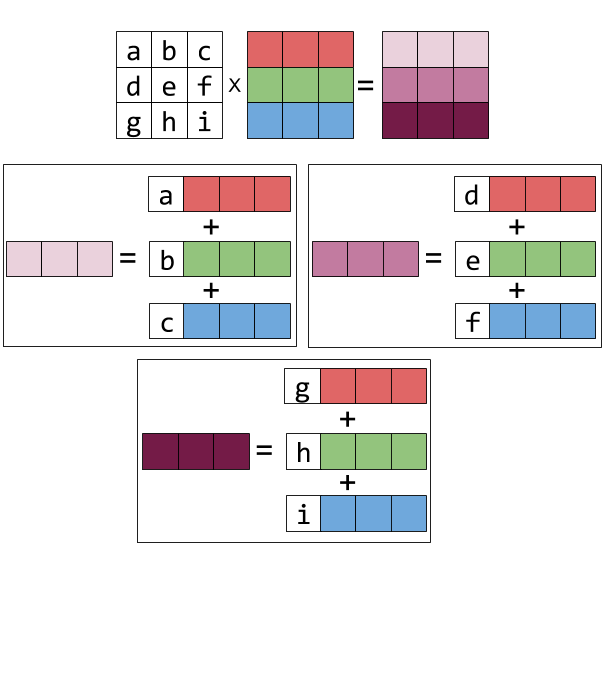Tags Math

When multiplying two matrices, there's a manual procedure we all know how to go through. Each result cell is computed separately as the dot-product of a row in the first matrix with a column in the second matrix. While it's the easiest way to compute the result manually, it may obscure a very interesting property of the operation: multiplying A by B is the linear combination of A's columns using coefficients from B. Another way to look at it is that it's a linear combination of the rows of B using coefficients from A.

In this quick post I want to show a colorful visualization that will make this easier to grasp.

## Right-multiplication: combination of columns

Let's begin by looking at the right-multiplication of matrix X by a column vector: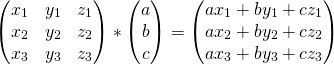Representing the columns of X by colorful boxes will help visualize this: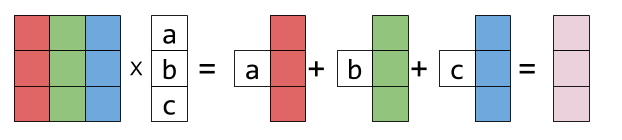Sticking the white box with a in it to a vector just means: multiply this vector by the scalar a. The result is another column vector - a linear combination of X's columns, with a, b, c as the coefficients.

Right-multiplying X by a matrix is more of the same. Each resulting column is a different linear combination of X's columns: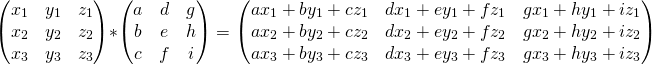Graphically:If you look hard at the equation above and squint a bit, you can recognize this column-combination property by examining each column of the result matrix.

## Left-multiplication: combination of rows

Now let's examine left-multiplication. Left-multiplying a matrix X by a row vector is a linear combination of X's rows: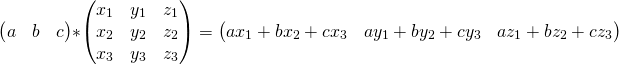Is represented graphically thus: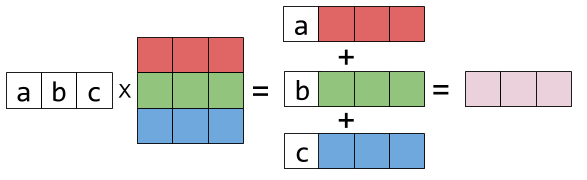And left-multiplying by a matrix is the same thing repeated for every result row: it becomes the linear combination of the rows of X, with the coefficients taken from the rows of the matrix on the left. Here's the equation form: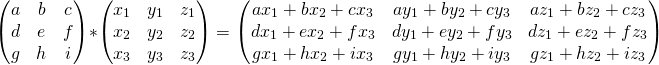And the graphical form: